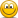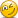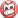# BODMAS remembered!!

#### Brian Hamilton

Level 5 Referee
Observer/Tutor
I wrote this yesterday and forgot to post it...

Hi, secondary maths teacher here. I'll try to get this rightThree pairs of shoes = 30. Therefore single shoe is 30/6 = 5
Two boys plus pair of shoes = 20. We have already established single shoe = 5, so pair of shoes = 10. This means two boys = 20 - 10.
So boy is 10/2 = 5
Four bags of chips plus a boy = 13. We have already established that a boy = 5. Therefore two pairs of double chips = 13 - 5.
So 4 bags of chips = 8 4. So single chips = 2

Now it's time to think about BIDMAS and Algebra.

BIDMAS (BODMAS)

Brackets - we have none
Indices (Orders) - we have none
Division and Multiplication are simultaneous but we only have Multiplication

Algebra - when we put two terms next to each other with no operation sign (add, subtract, multiply or divide) between them, they are deemed to have multiplied by each other.

So this should work out as

Shoe + boy x pair of shoes x two bags of chips x bag of chips

If we substitute the values back in, we get

5 + 5 x 10 x 4 x 2

and if we simplify this into the value for each symbol (shoe, dressed up boy with chips, chips) it becomes

= 5 + 200 x 2
= 5 + 400
= 405

#### Brian Hamilton

Level 5 Referee
Observer/Tutor
Final calculation should be:

5 + ((5 + 4 + 10) × 2) = 43

My brackets......nothing complex at all, its about identifying the middle person is wearing trainers and carrying two cones of chips( at least I think they're chips)
Items next to each other in algebra are multiplied together not added together.

•one

#### afronaut81

##### Well-Known Member
Level 7 Referee
I respect the length to which you've gone to do this and follow your logic. But:
If items next to each other are implied to be multiplied then it would stand that in the first row the left shoe and right shoe need to be multiplied together to make 10.

In that case the value of a shoe becomes unknown (unless it is the square root of 10) and the puzzle unsolvable.

I think we're over thinking a simple internet puzzle•Mr Dean

#### Sheffields Finest

##### Maybe I'm foolish, maybe I'm blind!
Level 7 Referee
In next weeks lesson homeschoolers, @Brian Hamilton will be teaching us the joys of Calculus for Idiots & What Women want...... Stay tuned!!!#### Brian Hamilton

Level 5 Referee
Observer/Tutor
I respect the length to which you've gone to do this and follow your logic. But:
If items next to each other are implied to be multiplied then it would stand that in the first row the left shoe and right shoe need to be multiplied together to make 10.

In that case the value of a shoe becomes unknown (unless it is the square root of 10) and the puzzle unsolvable.

I think we're over thinking a simple internet puzzleHa ha, you had me worried then but are so almost right.

Think of each shoe as represented by the letter S. Therefore each pair of shoes is not S squared but 2S. Otherwise 22 would also be 2 squared

We don't DO implied in MathsLast edited:
•afronaut81

#### one

Level 7 Referee
Final calculation should be:

5 + ((5 + 4 + 10) × 2) = 43

My brackets......nothing complex at all, its about identifying the middle person is wearing trainers and carrying two cones of chips( at least I think they're chips)
Ok you have identified the person has trainers and chips so you have to factor in 4 and 10 (assuming smaller image size is not a factor). What makes you add those numbers to 5 and not multiply them. That is, why not

5 + (5 x 4 x 10) x 2) = 405

There is nothing in the image saying they have to be added. In fact if you go by mathematical convention, when there is no operators between variables and coefficients, they have to be multiplied. For example if 'a=4' and 'Y=5' then 'aY=20'

I think you get my point now. I am not saying your answer is incorrect. Just saying it is not the only correct one.

EDIT. Just saw Brian's post. Oh well.

#### one

Level 7 Referee
I respect the length to which you've gone to do this and follow your logic. But:
If items next to each other are implied to be multiplied then it would stand that in the first row the left shoe and right shoe need to be multiplied together to make 10.

In that case the value of a shoe becomes unknown (unless it is the square root of 10) and the puzzle unsolvable.

I think we're over thinking a simple internet puzzleDouble win for the designer of the question#### Brian Hamilton

Level 5 Referee
Observer/Tutor
Ok you have identified the person has trainers and chips so you have to factor in 4 and 10 (assuming smaller image size is not a factor). What makes you add those numbers to 5 and not multiply them. That is, why not

5 + (5 x 4 x 10) x 2) = 405

There is nothing in the image saying they have to be added. In fact if you go by mathematical convention, when there is no operators between variables and coefficients, they have to be multiplied. For example if 'a=4' and 'Y=5' then 'aY=20'

I think you get my point now. I am not saying your answer is incorrect. Just saying it is not the only correct one.

EDIT. Just saw Brian's post. Oh well.
/tiphat to you @one

•one

#### Big Cat

Level 6 Referee
It's all nonsense then and has wasted 5 minutes of my precious time in which i could've been doing...... well... nothingLast edited:
••Mr Dean, Sheffields Finest and afronaut81

#### afronaut81

##### Well-Known Member
Level 7 Referee
"What did you do during the great pandemic granddad?"
"I debated math puzzles with referees"

••Tealeaf, JamesL, Mr Dean and 1 other person

#### afronaut81

##### Well-Known Member
Level 7 Referee
Algebra - when we put two terms next to each other with no operation sign (add, subtract, multiply or divide) between them, they are deemed to have multiplied by each other.
By that logic
Let shoe = S
Shoe next to shoe = S x S
= 10

Or we allow the two shoes to be different values in which case the puzzle is unsolvable

•Brian Hamilton

#### Brian Hamilton

Level 5 Referee
Observer/Tutor
By that logic
Let shoe = S
Shoe next to shoe = S x S
= 10

Or we allow the two shoes to be different values in which case the puzzle is unsolvable
Otherwise 22 would also be 2 squared
I rest my laptop

#### Mr Dean

##### Active Member
Think of each shoe as represented by the letter S. Therefore each pair of shoes is not S squared but 2S. Otherwise 22 would also be 2 squared
Huh? SS is S squared! And, 22 is NOT 2(2).

I think this lockdown might just improve your students' grades#### one

Level 7 Referee
The things we turn to argue about when we are deprived of arguing about football refereeing•Brian Hamilton and afronaut81

#### Sheffields Finest

##### Maybe I'm foolish, maybe I'm blind!
Level 7 Referee
Hold on, its just gone to VAR...............Leeds & Sydney may just have celebrated a bit early, @Brian Hamilton is an expert at this from memory!!#### Mintyref

Level 6 Referee
Items next to each other in algebra are multiplied together not added together.
That's if you look at them as single entities.....I look at the whole

•afronaut81

#### one

Level 7 Referee
But what does the spirit of the puzzle say? What does puzzle expect?

••Mr Dean, Russell Jones, Brian Hamilton and 1 other person

#### Mr Dean

##### Active Member
But what does the spirit of the puzzle say? What does puzzle expect?
It is usually 43.
Except for the above case, it is not usually 405.

•one and Sheffields Finest

#### Sheffields Finest

##### Maybe I'm foolish, maybe I'm blind!
Level 7 Referee
OK..... Enough of that, an easier one to calm the nerves!!

8/2(2+2)=